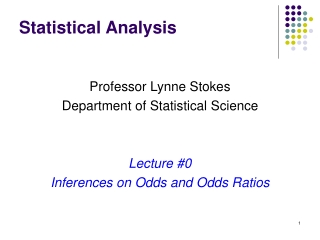DownloadDownload PresentationStatistical Analysis

Statistical Analysis

Télécharger la présentationStatistical Analysis

- - - - - - - - - - - - - - - - - - - - - - - - - - - E N D - - - - - - - - - - - - - - - - - - - - - - - - - - -
Presentation Transcript

1. Statistical Analysis Professor Lynne Stokes Department of Statistical Science Lecture #0 Inferences on Odds and Odds Ratios

2. Cardiovascular Deaths Among Obese Samoan Women:Odds Ratios Proportions 0.00776 0.00670 Controversy : Social stigma of being obese might be contributing to increased cardiovascular deaths, not obesity itself. In Samoa, there is no negative social effects of being obese.

3. X not sufficient

4. Cold Incidence

5. Difficulties with Proportions • Absolute differences can be interpreted differently depending on the magnitudes of the proportions • p1 – p2 = 0.05 when p1 = 0.90 and p2 = 0.85 (small relative change) • p1 – p2 = 0.05 when p1 = 0.10 and p2 = 0.05 (large relative change)

6. Odds and Odds Ratios • Customary to make Event 1 the larger of the odds • p = ½ corresponds to w = 1 • If odds of yes are w, odds of no are 1/ w • If odds of yes are w, probability of yes is w / (1 - w) Odds for a “success” Odds for a “failure” Ratio of the odds for “Success in Event 1” relative to odds for “Success in Event 2”

7. Odds and Odds Ratios

8. Incidence of Colds

9. Samoan Obesity Obese Odds : Odds of 128 to 1 against CVD death for an obese Samoan woman (1 / 0.007824) Not Obese Odds: Odds of 149 to 1 against CVD death for a nonobese Samoan woman (1 / 0.006705) Odds Ratio : Relative Odds of 1.2 to 1 for an obese Samoan woman to die of CVD than for a nonobese Samoan woman to die of CVD.

10. Inferences Use binomial inferences for directly comparing the population proportions Inferences on individual odds can be made from the proportion. compare odds using inferences on the odds ratio.

11. Confidence Intervals Testing Equality (f = 1 p1 = p2) If proportion unknown, replace with pooled estimate Asymptotic Distributions Note: Can also use inferences on binomial proportions – but not for retrospective studies.

12. Shortcut for Estimated SE for Confidence Intervals

13. Incidence of Colds

14. Retrospective Studies

15. Retrospective Studies Cold Study Samoan Obesity Smoking & Cancer Study

16. Invariance of Odds Ratio